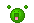# Fibonacci Sequence

16 views
If you want to know what the Fibonacci sequence is here is how you find it.

The Fibonacci sequence simply is: C=A+B here is an example: 1=+2=3 or 3+2=5

The Fibonacci sequence is: 0 1 1 2 3 5 8 13 21 34 55.......

so it=  1+1=2  2+1=3 3+2=5 5+2=8 8+5=13 13+8=21 21+13=34 34+21=55.........

this is the fibonacci sequence!

-jabber

That's is so cool!!!What grade are you in?

answered Nov 11 by (27,550 points)
7th
+1 vote
thanks!
answered Nov 11 by (366,280 points)